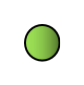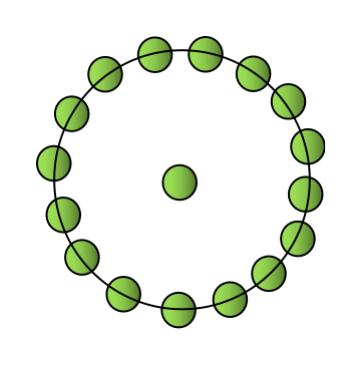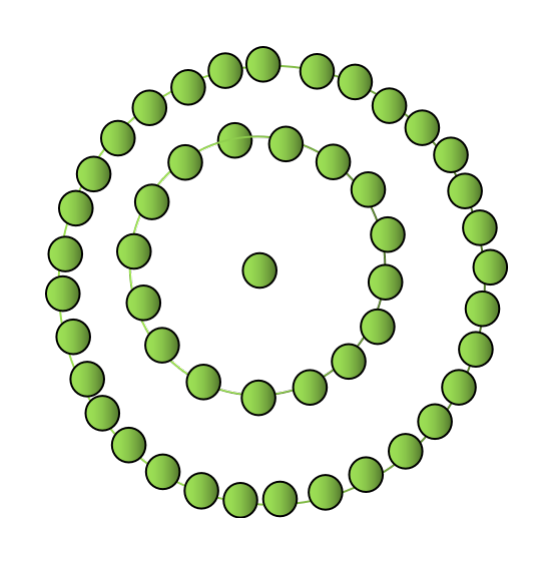Hexadecagonal numbers are the numbers that represent a hexadecagon. Hexadecagon is a polygon which consists of 16 sides.

A Centred Hexadecagonal number is the number represented by a dot in the centre and other dots surrounding it in the successive hexadecagonal layers i.e. 16 sided polygon. We can understand the centred hexadecagonal number better with the below figures.There is a single dot in the centre for the first centred hexadecagon number. So the first centred hexadecagonal number will be 1.There is a single dot followed by a hexadecagon surrounding it in the next centred hexadecagon number. So the next number will be 17.The next centred hexadecagon number will be 49 as there will be a single dot in the centre followed by successive hexadecagonal layers surrounding it i.e. 16 and 32. Thus the number will be 16+32+1=49.

The similar concept will be followed for every nth centred hexadecagon number. With the same reference, the first few centred hexadecagonal number are 1, 17, 49, 97, 161, 241……

The problem statement includes that we need to print the Nth centred hexadecagonal number for the given input N.

For example,

INPUT : 5

OUTPUT : 161

INPUT: 8

OUTPUT: 449

Below is the algorithm to solve the problem.

## Algorithm

For this problem, we need to identify the pattern that is being followed in the problem in order to calculate the nth centred hexadecagonal number.

The concept of centred hexadecagonal number says that it is represented by a dot in the centre and successive layers of hexadecagon surrounding it. The successive hexadecagon layers can be represented as 16, 32, 48, 64…. If we pay attention to the pattern formed, it is forming an A.P. with a common difference of 16.

As the first few centred hexadecagon numbers are 1, 17, 49, 97…. If we closely observe the pattern, it is nothing but the sum of successive hexadecagonal layers up to N starting with 0, and 1.

The sequence of successive hexadecagonal layers starting with 0 will be 0, 16, 32, 48, 64….

• The 1st centred hexadecagonal number is 1 which is 0+1.

• The 2nd centred hexadecagonal number is 17 which is 0+16+1.

• The 3rd centred hexadecagonal number is 49 which is 0+16+32+1.

• The 4th centred hexadecagonal number is 97 which is nothing but the sum of successive hexadecagonal layers up to N(which is 4) and 1. It can be represented as 0+16+32+48+1.

With the above examples, we can consider that the nth centred hexadecagonal number is the sum of an A.P. of n terms whose common difference is 16 and first term is 0. To calculate the nth term of centred hexadecagonal number, it can be written as −

CHn= sum of n terms of an ap + 1

$$\mathrm{CH_n\:=\:\frac{n}{2}(2a\:+(n-1)d)+1}$$

a= first term of the A.P. which is 0

d= common difference of the A.P. i.e. 16

Putting the required values in the above formula, it can be further modified as −

$$\mathrm{CD_n\:=\:\frac{16n}{2}(n-1)+1}$$

$$\mathrm{CD_n\:=\:8n(n-1)+1}$$

We will be using the above derived formula to calculate the nth centred hexadecagonal number in our approach.

## Approach

• Initialise a function to calculate the Nth centred hexadecagonal number.

• Use the above derived formula to calculate the Nth value.

• We will initialise a variable to store the value of the nth centred hexadecagonal number.

• Return the variable which will be our required output.

## Example

Below is the implementation of the approach in C++ −

#include <iostream>
#include<bits/stdc++.h>

using namespace std;

//function to calculate the nth centred Hexadecagonal number
int CHn(int N){
int a= 8 * N * (N-1) + 1; //used to store nth centred Hexadecagonal number value

}
int main(){
int N=5;
cout<<CHn(N)<<endl;

N=10;
cout<<CHn(N)<<endl;

N=13;
cout<<CHn(N)<<endl;

return 0;
}


## Output

161
721
1249


Time Complexity : O(1) , because constant time is taken.

Space Complexity : O(1) , because no extra space is required.

## Conclusion

We have tried to learn the concept of centred hexadecagonal number in this article. We also come up with an approach to print the Nth centred hexadecagonal number for any positive number N.